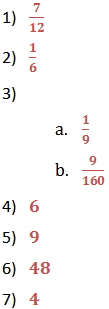Multiply Fractions and Mixed Numbers

Multiply Fractions and Mixed Numbers

Multiply Fractions

To multiply fractions by fractions, multiply the numerators, and then multiply the denominators. Once you have the product, see if it can be reduced to the simplest form.

It is often helpful to cancel out common factors from the numerator and denominator before multiplying.

Different ways to find  of

 Multiply, then simplify Simplify, then multiply

Multiply Fractions by Whole Numbers and Mixed Numbers

To multiply a whole number by a fraction, first write the whole number with a denominator of 1.  Then multiply the fractions by multiplying the numerators and denominators across.  Reduce to simplest form.

To multiply a mixed number by a fraction, or to multiply two mixed numbers, write any mixed number as an improper fraction.  Then multiply the fractions.

For example solve the following expression: 3 2

First change mixed numbers to improper fractions

3 2 =

Then multiply numerators and denominators

Write the final expression as a mixed number and simplify

9 = 9

Try it yourself

Find each product.

1.)

2.)

3a.)

3b.)

4.) 8

5.) 3 2

6.) 8 5

7.) 1 2